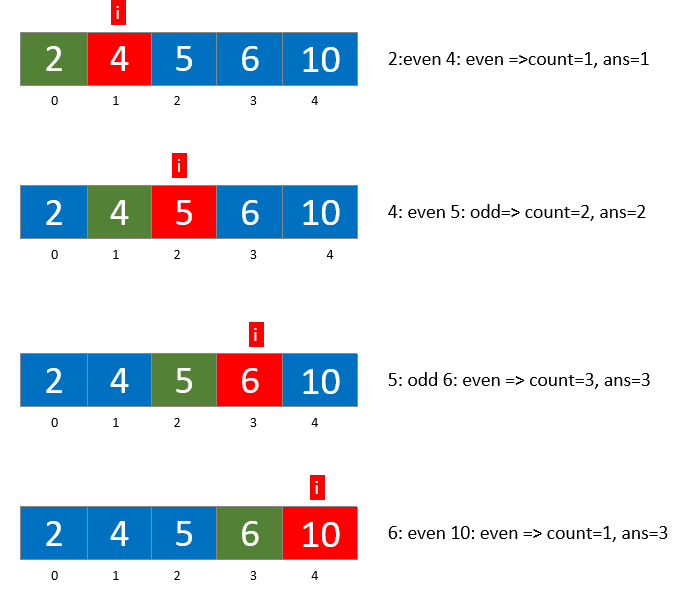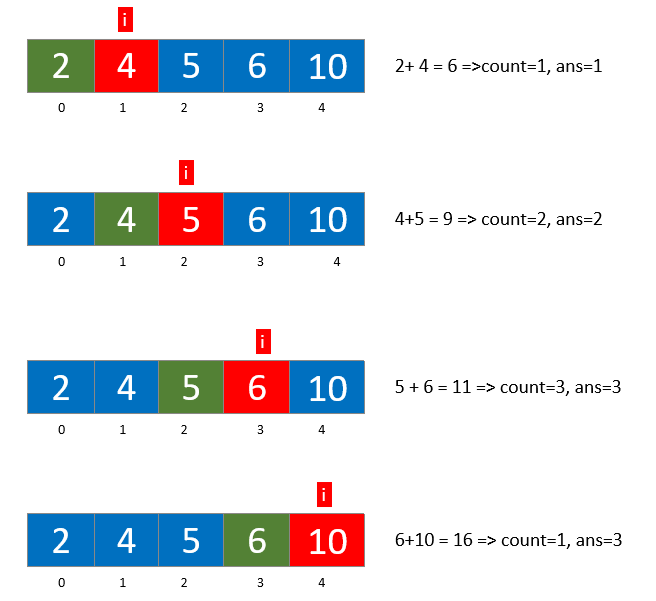# Longest Even Odd Subarray

Problem Statement: Given an array of N integers, find the length of the longest alternating even-odd subarray present in the array.

Examples:

```Example 1:
Input: arr[]={1, 2, 3, 4, 5, 7, 9}
Output: 5
Explanation: The longest subarray with alternate even odd subarray is {1,2,3,4,5}

Example 2:
Input: arr[]={2,3,4,6,10}
Output: 3
Explanation: The longest subarray with alternate even odd subarray is {2,3,4}```

### Solution:

DisclaimerDon’t jump directly to the solution, try it out yourself first.

### Naive Approach:

In this approach, we find the longest even-odd or odd-even subarray for every element and later find the maximum length of them.

We iterate through the array and for every element we again iterate through the array to find the subarray size. Later we find the maximum length out of

The following code explains this approach in a better way

Code:

## C++ Code

``````#include <iostream>
using namespace std;

void evenodd_naive(int arr[], int size) {
int ans = 0;
for (int i = 0; i < size; i++) {
int count = 1;
for (int j = i + 1; j < size; j++) {
if ((arr[j - 1] % 2 == 0 && arr[j] % 2 != 0) || (arr[j - 1] % 2 != 0 && arr[j]
% 2 == 0)) {
count++;
} else break;
}
ans = max(ans, count);
}
cout <<"The length of the longest even-odd subarray is "<<ans << "\n";
}
int main() {
int arr[] = {1,2,3,4,5,7,9};
int size = 7;
evenodd_naive(arr, size);
return 0; }
``````

Output: The length of the longest even-odd subarray is 5

Time complexity: O(n^2)

Space complexity: O(1)

## Java Code

``````public class Main {
static void evenodd_naive(int arr[]) {
int ans = 0;
for (int i = 0; i < arr.length; i++) {
int count = 1;
for (int j = i + 1; j < arr.length; j++) {
if ((arr[j - 1] % 2 == 0 && arr[j] % 2 != 0) || (arr[j - 1] % 2 != 0 && arr[j]
% 2 == 0)) {
count++;
} else break;
}
ans = Math.max(ans, count);
}

System.out.println("The length of the longest even-odd subarray is "+ans);
}

public static void main(String args[]) {
int arr[] = {1,2,3,4,5,7,9};
evenodd_naive(arr);
}
}
``````

Output: The length of the longest even-odd subarray is 5

Time complexity: O(n^2)

Space complexity: O(1)

### Approach 2: Kadane’s Algorithm

We can use kadane’s algorithm to find the solution to this problem. There are two possibilities:

1. We can extend the subarray when we have alternating even-odd or odd-even elements.
2. We jump to a new subarray when the above condition fails.

We can start our iteration from the first element and check if the previous element is odd and the current element is even and vice-versa. If this is true we increment the size of the subarray else we re-initialize the size of the subarray to 1. With every iteration, we continue to find the maximum size so as to prevent the usage of any data structure to store the lengths thus increasing the time complexity.

The following diagram explains the approach we are using hereCode:

## C++ Code

``````#include <iostream>
using namespace std;

void evenodd_kadane(int arr[], int size) {
int ans = 0;
int count = 1;
for (int i = 1; i < size; i++) {
if ((arr[i - 1] % 2 == 0 && arr[i] % 2 != 0) || (arr[i - 1] % 2 != 0 && arr[i]
% 2 == 0)) { // extending the subarray
count++;
ans = max(ans, count);
} else count = 1; // choosing the new subarray
}
cout <<"The length of the longest even-odd subarray is " <<ans << "\n";
}
int main() {
int arr[] = {1,2,3,4,5,7,9};
int size = 7;
return 0; }
``````

Output: The length of the longest even-odd subarray is 5

Time complexity: O(n)

Space complexity: O(1)

## Java Code

``````public class Main {
static void evenodd_kadane(int arr[]) {
int ans = 0;
int count = 1;
for (int i = 1; i < arr.length; i++) {
if ((arr[i - 1] % 2 == 0 && arr[i] % 2 != 0) || (arr[i - 1] % 2 != 0 && arr[i]
% 2 == 0)) { // extending the current subarray
count++;
ans = Math.max(count, ans);
} else count = 1; // choosing the new subarray
}
System.out.println("The length of the longest even-odd subarray is "+ans);
}

public static void main(String args[]) {
int arr[] = {1,2,3,4,5,7,9};
}
}
``````

Output: The length of the longest even-odd subarray is 5

Time complexity: O(n)

Space complexity: O(1)

### Approach 3: Simple Mathematics and Kadane’s Algorithm

Everyone knows that the sum of two even/odd numbers is even but the sum of an even and an odd number is odd.

We iterate through the array starting from the 2nd element and check if the sum of the previous element and current element is odd, we increment the size of the subarray, or else we choose the new subarray.

This approach is very similar to our second approach.

The following diagram explains the above approachCode:

## C++ Code

``````#include <iostream>
using namespace std;

void evenodd_mathKadane(int arr[], int size) {
int ans = 0;
int count = 1;
for (int i = 1; i < size; i++) {
if ((arr[i - 1] + arr[i]) % 2 != 0) { // extending the same subarray
count++;
ans = max(ans, count);
} else count = 1; // choosing the new subarray
}
cout <<"The length of the longest even-odd subarray is "<<ans;
}
int main() {
int arr[] = {1,2,3,4,5,7,9};
int size = 7;
return 0;
}
``````

Output: The length of the longest even-odd subarray is 5

Time complexity: O(n)

Space complexity: O(1)

## Java Code

``````
public class Main {
static void evenodd_mathKadane(int arr[]) {
int ans = 0;
int count = 1;
for (int i = 1; i < arr.length; i++) {
if ((arr[i - 1] + arr[i]) % 2 != 0) { // extending the same subarray
count++;
ans = Math.max(ans, count);
} else count = 1; // choosing the new subarray
}
System.out.println("The length of the longest even-odd subarray is "+ans);
}
public static void main(String args[]) {
int arr[] = {1,2,3,4,5,7,9};